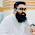## 2013年8月10日

### UVa 11456 - Trainsorting

```#include <cstdio>

int main() {
int T;
scanf("%d", &T);
while (T--) {
int n, w;
scanf("%d", &n);
int lUp, lDown;
for (int i = 0; i < n; i++) {
scanf("%d", &w[i]);
lUp[i] = lDown[i] = 1;
}
int max = 0;
for (int i = n - 1; i >= 0; i--) {
for (int j = i + 1; j < n; j++) {
if (w[j] > w[i] && lUp[j] + 1 > lUp[i]) {
lUp[i] = lUp[j] + 1;
}
if (w[j] < w[i] && lDown[j] + 1 > lDown[i]) {
lDown[i] = lDown[j] + 1;
}
}
int sum = lUp[i] + lDown[i] - 1;
max = sum > max ? sum : max;
}
printf("%d\n", max);
}
return 0;
}
```

#### 1 則留言:

1.why you started calculating LIS from n-1 instead of 0 index.
This algo is not working when I start from index 0.